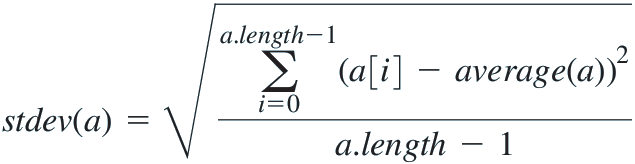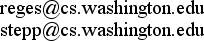## Building Java Programs: A Back to Basics Approach, 5th edition by Stuart Reges and Marty Stepp

Authors' official companion web site

## Errata, 4th Edition

• p69, Chapter 2 - The code sample is missing a minus ( `-` ) sign. It should be:
`x % y = x - (x / y) * y`
• p92, Chapter 2 - The code sample is missing a minus ( `-` ) sign. It should be:
`for (int i = -100; i <= 100; i++) {`
• p95, Chapter 2 - The syntax template shows a variable `i` where it should say `<variable>`. It should be:
`for (int <variable> = 1; <variable> <= n; i++) {`
`for (int <variable> = 1; <variable> <= n; <variable>++) {`
• p96, Chapter 2 - The syntax template shows an elongated dash (—) when it should show two minus signs ( `--` ). It should be:
`for (int <variable> = n; <variable> >= 1; <variable>--) {`
• p114, Chapter 2 - Three lines in the code sample are missing minus ( `-` ) signs. They should be:
```line 41:    for (int i = 1; i <= (3 - line); i++) {
line 45:    for (int i = 1; i <= 2 * (line - 1); i++) {
line 49:    for (int i = 1; i <= (3 - line); i++) {
```
• p117, Chapter 2 - Line 52 of the code sample is missing a minus ( `-` ) sign. It should be:
`for (int i = 1; i <= (SUB_HEIGHT - line); i++) {`
• p124, Chapter 2 - Two of the self-check problem's answer choices are missing minus ( `-` ) signs. They should be:
• c. `30, 20, 10, 0, -10, -20, . . .`
• d. `-7, -3, 1, 5, 9, 13, . . .`
• p167, Ch. 3: The table text:
`s.replace("l", "y") returns "heyyyyo"`
`s.replace("l", "yy") returns "heyyyyo"`
(thanks to James Kim of Bellevue College, future CTO)
• p378, Ch. 5: Exercise 5.4 `randomX` has a small typo. It says "prints a lines" when it should say "prints lines".
• p379, Ch. 5: Exercise 5.9 says at the end, "Your method should print nothing if the empty string (`""`) is passed." But this text does not make sense for this problem, because the method accepts an `int` parameter, not a string. This text should be disregarded. (thanks to Richie Sweeney)
• p523, Ch. 7: Exercise 7.6 `stdev` has the wrong formula for the standard deviation due to a printing error. The formula should be:(thanks to James Livingston)
• p549, Ch. 8: The text, "A `Point` reference variable named `p` is created," should read, "A `Point` reference variable named `p1` is created." (thanks to Timur Rikhsiev)
• p805, Ch. 12: In the code for the `explore` method, there is a missing right curly brace just before the line that says "return false". The complete code sample should be:
```private static boolean explore(Board b, int col) {
if (col > b.size()) {
return true;
} else {
for (int row = 1; row <= b.size(); row++) {
if (b.isSafe(row, col)) {
b.place(row, col);
if (explore(b, col + 1)) {
return true;
}
b.remove(row, col);
}
}
return false;
}
}
```
(Thanks to Cheng Xing)

### Reporting Errors:

Have you found an error in our textbook? If so, please email us at: